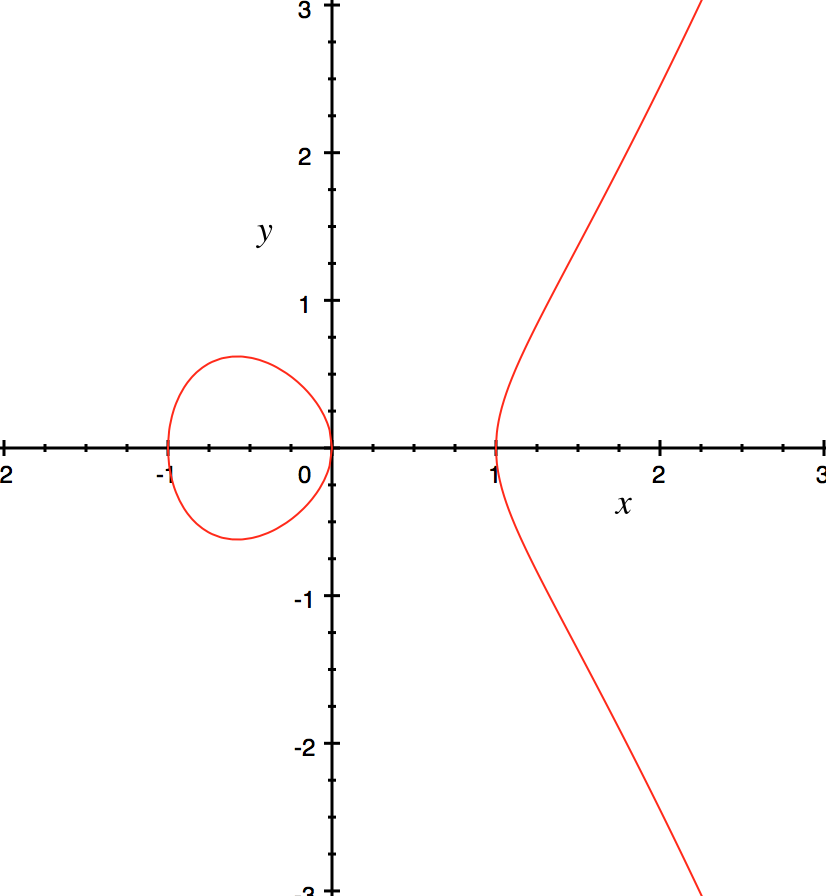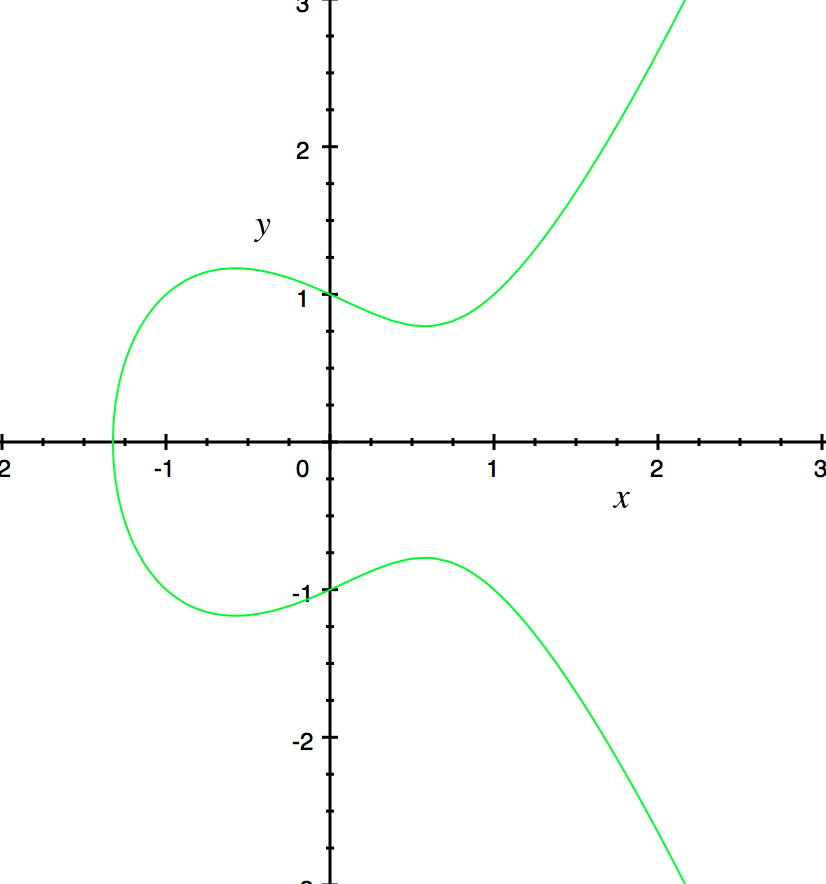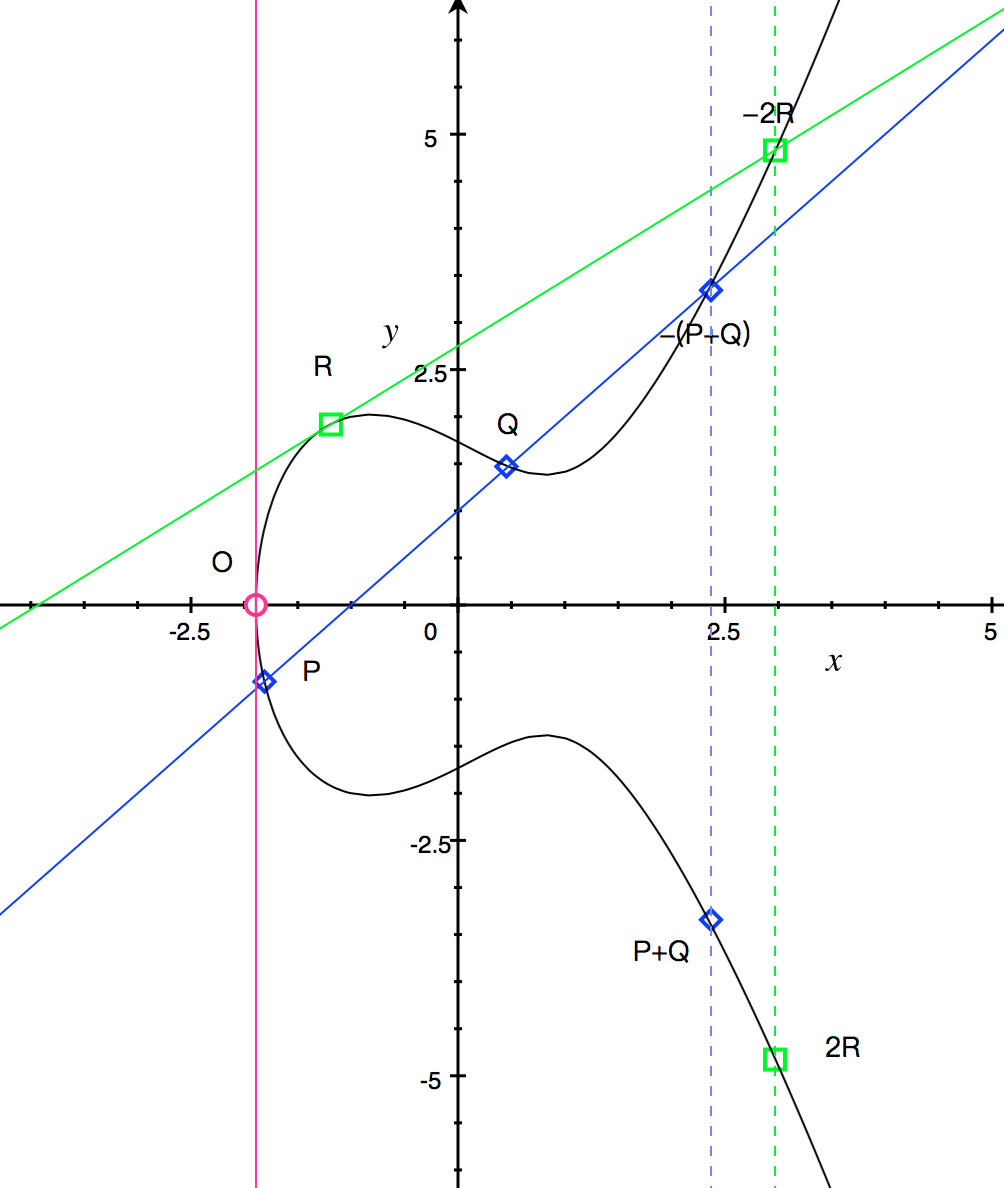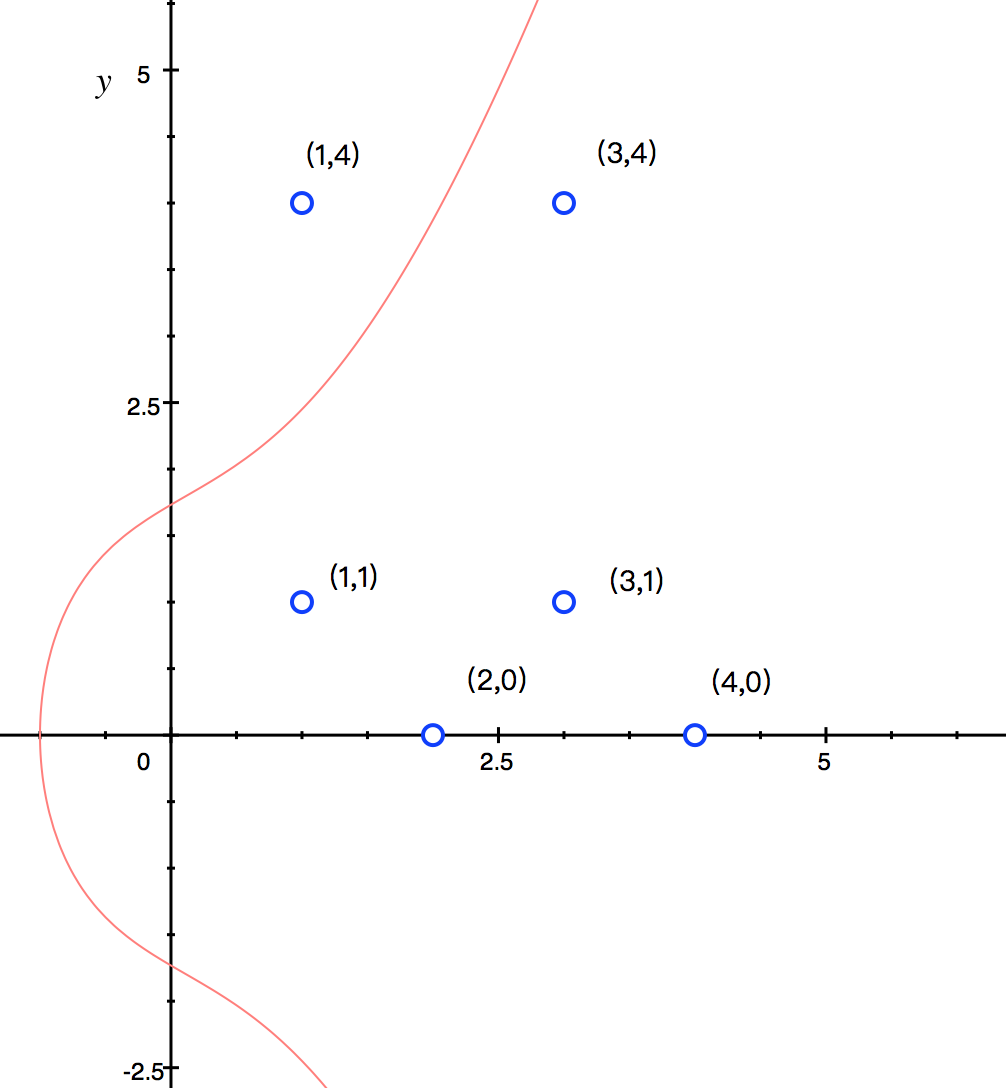# secp256k1⌗

secp256k1是由(Standards for Efficient Cryptography)定制的一种椭圆曲线签名算法，在比特币流行以前，该算法几乎没有被使用过。大多数常用的曲线具有一个随机的结构，但是secp256k1是为了更有效率的计算而构造了一个非随机结构。因此如果该算法的实现通过合理的优化，其计算效率可以比别的曲线快30%以上。同时，与常用的NIST曲线不同，secp256k1的常量是通过可预测的方式挑选的，这可以有效的减少防止曲线设计者安置后门的可能性。具体规范可以参考Secp256k1SEC-V2

### 椭圆曲线签名算法⌗

1. Alice选择一个随机自然数$a$，然后将$g^a$发送给Bob

2. Bob选择一个随机自然数$b$，然后将$g^b$发送给Alice

3. Alice计算$(g^{b} )^a$

4. Bob计算$(g^{a} )^b$

### 椭圆曲线的数学描述⌗

a. 曲线 $y^2 =x^3 -x$b. 曲线 $y^2 =x^3 -x +1$1. 对于$\mathcal{O}$，满足 $$\mathcal{O}+\mathcal{O}=\mathcal{O}$$
2. 对于椭圆曲线上任意一点$$(x,y)+ \mathcal{O} = \mathcal{O} + (x,y) = (x,y)$$
3. 对于任意点$(x,y)$，存在一个逆元$(x,-y)$，使得$$(x,y)+(x,-y)=\mathcal{O}$$
4. 对于任意x轴不同的两点$(x_1,y_1)$、$(x_2,y_2)$，可以构建$$(x_1,y_1)+(x_2,y_2)=(x_3,y_3)$$ 其中$x_3=\lambda^2 -x_1-x_2$，$y_3=\lambda(x_1-x_3)-y_1$，$\lambda=(y_2-y_1)/(x_2-x_1)$
5. 对于任意一点$(x,y)$，可以对自身做加法，使得$$(x,y)+(x,y)=2(x,y)=(x_3+y_3)$$ 其中$x_3=\lambda^2 -2x$，$y_3=\lambda(x-x_3)-y$，$\lambda=(3x^2+a)/(2y)$
##### 椭圆曲线加法的几何意义⌗1. 蓝色线为普通的相交线，和椭圆曲线相交于三个点$P,Q,-(P+Q)$，三者之和为$P+Q+(-(P+Q))=\mathcal{O}$

2. 绿色线为切线，和椭圆曲线切于点$R$，交于$-2R$，切点当作两点，三者之和为$R+R+(-2R)=\mathcal{O}$

3. 粉色线为特殊切线，和椭圆曲线切于一个点，别的点不相交，则该点$O$可以被定义为$O=\mathcal{O}$

4. 蓝色和绿色虚线均和椭圆曲线交于两点，分别的可以得到$(P+Q)+(-(P+Q))=\mathcal{O}$和$(2R)+(-2R)=\mathcal{O}$

### 有限域上的椭圆曲线⌗

##### 有限域$\mathcal{F}_p$上的椭圆曲线⌗1. $\mathcal{O}=(4,0)$，$\mathcal{O}+\mathcal{O}=\mathcal{O}$，满足定义1。

2. $P=(1,1),Q=(1,4)$，则$P+Q=\mathcal{O}$。注意到$4\equiv -1\bmod{5}$，符合定义3。

3. $P=(1,1),Q=(2,0)$，则$P+Q=(3,1)$。符合定义4。

4. $P=(1,1)$，则$P+P=2P=(3,4)$。符合定义5。

##### 椭圆曲线签名算法密码交换举例⌗

1. Alice选择一个随机自然数$a=2$，然后将$g^{a}=2*g=(3,4)$发送给Bob

2. Bob选择一个随机自然数$b=3$，然后将$g^{b}=3*g=(2,0)$发送给Alice

3. Alice计算 $(g^b )^{a}=2*(2,0)=(4,0)$

4. Bob计算 $(g^a )^{b}=3*(3,4)=(4,0)$

##### 椭圆曲线数字签名算法(ECDSA)⌗

1. 随机生成密钥对$(k, K)$，其中$K=(K_x, K_y)$

2. 令$r = K_x \bmod n$，如果$r = 0$，则返回步骤1

3. 计算$H = Hash(M)$，按照数据类型转换规则，将$H$转化为一个大端整数$e$

4. 令$s = k^{-1} (e + rd) \bmod n$，若$s = 0$, 则返回步骤1

5. 输出的$Signature(M)=(r,s)$即为签名。

1. 计算$H = Hash(M)$，按照数据类型转换规则，将$H$转化为一个大端整数$e$

2. 计算 $u_1 = s^{-1} e \bmod n$, $u_2 = s^{-1} r \bmod n$

3. 计算 $K=(K_x, K_y) = u_1 G + u_2 D$, 如果 $K = \mathcal{O}$，则验证该签名无效

4. 计算 $v = K_x \bmod n$，若$v = r$，则签名有效，否则签名无效。